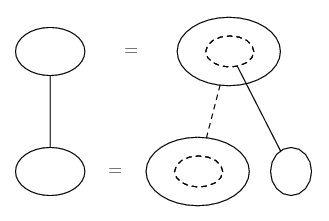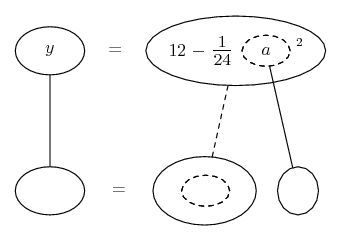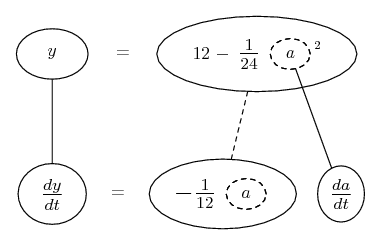# Thread: Related Rates

1. ## Related Rates

A winch at the top of a 12 m building pulls a pipe of the same length to a vertical position. The winch pulls the rope at a rate of -0.2 meters per second. find the rate of vertical change and the rate of horizontal change at the end of the pipe when y=6.

2. Hello, rawkstar!

The directions are not clear.
I assume that problem came with a labeled diagram.

A winch at the top of a 12 m building pulls a pipe of the same length to a vertical position.
The winch pulls the rope at a rate of -0.2 meters per second.
Find the rate of vertical change and the rate of horizontal change at the end of the pipe when $\displaystyle y=6$.
First of all, what is $\displaystyle y$ ?

And are they asking for the changes at the ends of the pipe?

I'm trying to envision the problem.

The pipe is lying horizontally on the ground, perpendicular to the wall of the building..
The rope is attached to the near end of the pipe.
Code:
          W
- - *   *
|   :
|   :
|   :
12 |   :r
|   :
|   :
|   :
- - * - o * * * * * * o - -
A     12      B
The winch is at $\displaystyle W.$
The pipe is $\displaystyle AB = 12$.
The length of the rope is $\displaystyle r$.

As the rope gets shorter,
. . the top of the ladder $\displaystyle (A)$ is raised straight up,
. . and the bottom of ladder $\displaystyle (B)$ approaches the building.

Code:
          W
- - *   *
|   :
|   :r
|   :
12 |   o A
|   : *
|  y:   *
|   :     *
- - * - * - - - o - -
C   x   B
I would guess that we are concerned with $\displaystyle \frac{dy}{dt}$ and $\displaystyle \frac{dx}{dt}$

3. I think the rope is attached to the end of the pipe which is away from the wall, so that it makes sense to speak of the x and y movements of a single end. The only problem is that I think the wind is going to swing the pipe out of the x-y plane!

Anyway, the wall and the pipe and the rope make a gradually narrowing isosceles, so that we can apply the cosine rule to get the corner A at the ground in terms of a the length of the rope which is the side opposite A.

$\displaystyle \cos A = \frac{b^2 + c^2 - a^2}{2bc}$

$\displaystyle A = \arccos (\frac{b^2 + c^2 - a^2}{2bc})$

$\displaystyle = \arccos (1 - \frac{a^2}{288})$

Then if theta is the angle between the pipe and the ground,

$\displaystyle \theta = \arcsin (1 - \frac{a^2}{288})$

and the height of the end, y

$\displaystyle = 12 * \sin(\arcsin (1 - \frac{a^2}{288}))$

$\displaystyle = 12 (1 - \frac{a^2}{288}))$

Now the calculus to get dy/dt is easy enough - dx/dt looks nastier, though I'm probably missing a trick, so I'll do it after! (If someone else hasn't.)

Related rates nearly always depend on the chain rule, so we might want to try filling up this pattern...... where straight continuous lines differentiate downwards (integrate up) with respect to the main variable (in this case time), and the straight dashed line similarly but with respect to the dashed balloon expression (the inner function of the composite which is subject to the chain rule).

So if we express y as a function of a...then we can differentiate both sides with respect to t...

Spoiler:Now, we have a value we can sub in directly for da/dt. We'll have to solve to find a when y is 6, so perhaps it would have been better to express a as a function of y in the first place... though not a critical matter. Anyway, we can now get dy/dt. Time to investigate the x variation?

$\displaystyle x = 12 * \cos(\arcsin (1 - \frac{a^2}{288}))$

$\displaystyle = 12 * \sqrt{1 - (1 - \frac{a^2}{288})^2}$

Differentiating this may be a bit more challenging - having to apply the chain rule at least twice. Will have to leave it with you, I'm afraid (till tommorrow). Hope the whole idea wasn't a wrong turn...

__________________________________________

Don't integrate - balloontegrate!

Balloon Calculus: Gallery

Balloon Calculus Drawing with LaTeX and Asymptote!

rates, related